## Math 480 Course Notes -- June 5, 1996

### From Random Walk to Diffusion - a stochastic process continuous in both space and time

This time we will give a (first) derivation of the Fokker-Planck equation, which governs the evolution of the probability density function of a random variable-valued function X(t) that satisfies a "first-order stochastic differential equation". The variable evolves according to what in the literature is called a Wiener process, named after Norbert Wiener, who developed a great deal of the theory of stochastic processes during the first half of this century.

We shall begin with a generalized version of random walk (an "unbalanced" version where the probability of moving in one direction is not necessarily the same as that of moving in the other). Then we shall let both the space and time increments shrink in such a way that we can make sense of the limit as bothand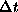approach 0.

Generalized random walk

We consider a generalized one-dimensional random walk (on the integers) beginning at X=0. We fix a parameter p, and let p be the probability of taking a step to the right, so that q=1-p is the probability of taking a step to the left, at any (integer-valued) time t. In symbols, this is: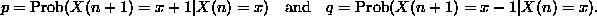Since we are given that X(0)=0, we know that the probability function of X(n) will be essentially binomially distributed (with parameters n and p, except that at time n, x will go from -n to +n, skipping every other integer as discussed earlier). We will set. Then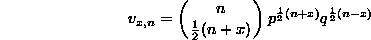for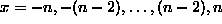and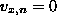otherwise.

For use later, we calculate the expected value and variance of X(n). First,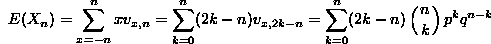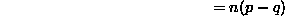(it's an exercise to check the algebra; but the result is intuitive). Also,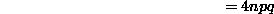(another exercise!). Also, note that we could have thought of X(n) as the sum of n independent binomially distributed random variables each having expected value p-q and variance 4pq. The same results follow from this observation.

Now, we let the space and time steps be different from 1. First, the time steps (that's easier). We will take time steps of length. This will have little effect on our formulas, except everywhere we will have to note that the number n of time steps needed to get from time 0 to time t is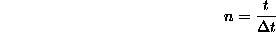For X, we change our notation so that each step to the left or right has lengthinstead of length 1. This has the effect of scaling X(t) by the factor. Multiplying a random variable by a constant has the effect of multiplying its expected value by the same constant, and its variance by the square of the constant. Therefore, the mean and variance of the total displacement in time t are: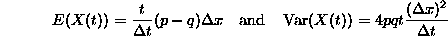We want both of these quantities to remain finite for all fixed t, since we don't want our process to have a positive probability of zooming off to infinity in finite time, and we also want Var(X(t)) to be non-zero. Otherwise the process will be completely deterministic.

We need to examine what we have control over in order to achieve these ends. First off, we don't expect to letandto go to zero in any arbitrary manner. In fact, it seems clear that in order for the variance to be finite and non-zero, we should insist thatbe roughly the same order of magnitude as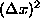. This is because we don't seem to have control over anything else in the expression for the variance. It is reasonable that p and q could vary with, but we probably don't want their product to approach zero (that would force our process to move always to the left or always to the right), and it can't approach infinity (since the maximum value of pq is 1/4). So we are forced to make the assumption thatremain bounded as they both go to zero. In fact, we will go one step further. We will insist that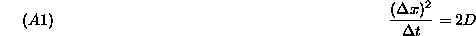for some (necessarily positive) constant D.

Now, we are in a bind with the expected value. Under assumption A1, the expected value will go to infinity as, and the only parameter we have to control it is p-q. We need p-q to have the same order of magnitude asin order to keep E(X(t)) bounded. Since p=q when p=q=1/2, we will make the assumption that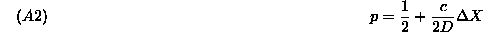for some real (positive, negative or zero) constant c, which entailsThe reason for the choice of the parameters will become apparent. D is called the diffusion coefficient, and c is called the drift coefficient.

Note that asandapproach zero, the values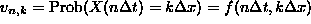specify values of the function f at more and more points in the plane. In fact, the points at which f is defined become more and more dense in the plane asandapproach zero. This means that given any point in the plane, we can find a sequence of points at which such an f is defined, that approach our given point asandapproach zero.

We would like to be able to assert the existence of the limiting function, and that it expresses how the probability of a continuous random variable evolves with time. We will not quite do this, but, assuming the existence of the limiting function, we will derive a partial differential equation that it must satisfy. Then we shall use a probabilistic argument to obtain a candidate limiting function. Finally, we will check that the candidate limiting function is a solution of the partial differential equation. This isn't quite a proof of the result we would like, but it touches on the important steps in the argument.

We begin with the derivation of the partial differential equation. To do this, we note that for the original random walk, it was true thatsatisfies the following difference equation: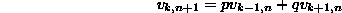This is just a specification of the matrix of the (infinite discrete) Markov process that defines the random walk. When we pass into the,realm, and use f(x,t) notation instead of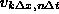, this equation becomes:Next, we need Taylor's expansion for a function of two variables. As we do the expansion, we must keep in mind that we are going to let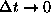, and as we do this, we must account for all terms of order up to and including that of. Thus, we must account for bothandterms (but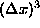and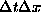both go to zero faster than).

We expand each of the three expressions in equation (*) around the point (x,t), keeping terms up to orderand. We get: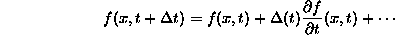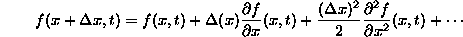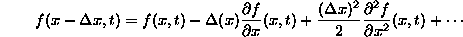We substitute these three equations into (*), recalling that p+q=1, and get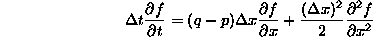(where all the partial derivatives are evaluated at (x,t)). Finally, we divide through by, and recall that, andto arrive at the equation:This last partial differential equation is called the Fokker-Planck equation.

To find a solution of the Fokker-Planck equation, we use a probabilistic argument to find the limit of the random walk distribution asandapproach 0. The key step in this argument uses the fact that the binomial distribution with parameters n and p approaches the normal distribution with expected value np and variance npq as n approaches infinity. In terms of our assumptions about,, p and q, we see that at time t, the binomial distribution has mean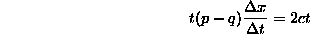and variancewhich approaches 2Dt as. Therefore, we expect X(t) in the limit to be normally distributed with mean 2ct and variance 2Dt. Thus, the probability thatis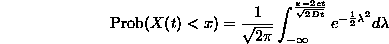Therefore the pdf of X(t) is the derivative of this with respect to x, which yieldsHomework

1. Verify that the expected value and variance of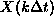are as claimed in the text.

2. Verify that the function f(x,t) obtained at the end of the section satisfies the Fokker-Planck equation.

Dennis DeTurck
Mon Jun 10 17:15:56 EDT 1996